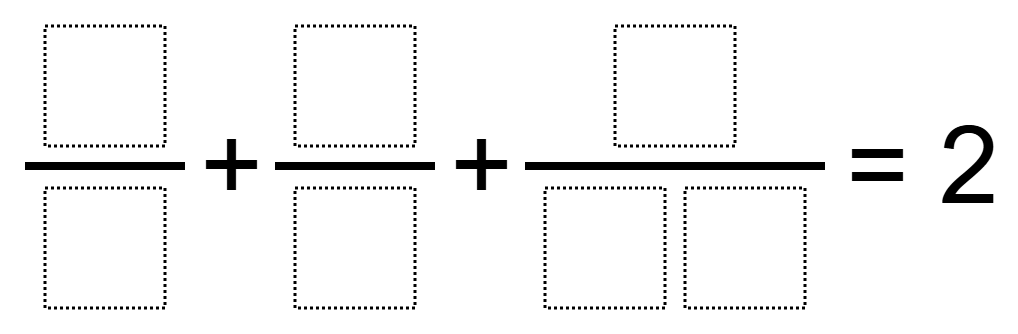Home > Grade 5 > Fractions: Sum of 2

# Fractions: Sum of 2

Directions: Use the digits 0 to 9, at most one time each, to fill in the boxes so that the sum is equal to 2 wholes.### Hint

What fractions are greater than 1 whole?
What manipulatives could help you find a solution?

There are many answers including:

9/6 + 1/4 + 5/20
6/5 + 4/8 + 3/10
4/5 + 6/8 + 9/20

Source: Joshua Nelson

## Multiplying Decimals to Make a Whole Number Product

Directions: Using the digits 1 to 9, at most one time each, fill in the …

### One comment

1.3/4 + 6/8 + 5/10 = 2